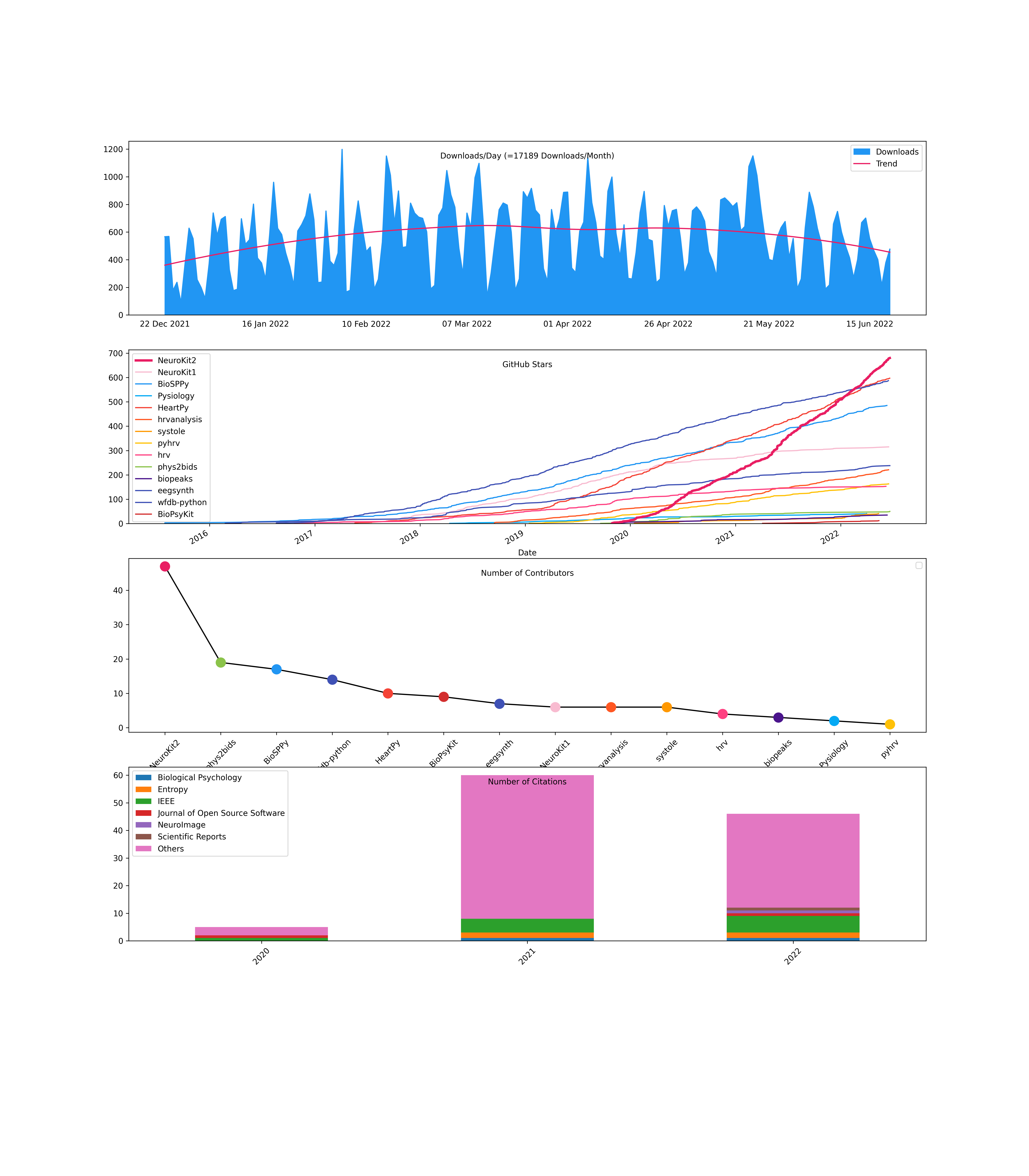# Introduction¶The Python Toolbox for Neurophysiological Signal Processing

NeuroKit2 is a user-friendly package providing easy access to advanced biosignal processing routines. Researchers and clinicians without extensive knowledge of programming or biomedical signal processing can analyze physiological data with only two lines of code.

## Quick Example¶

```import neurokit2 as nk

data = nk.data("bio_eventrelated_100hz")

# Preprocess the data (filter, find peaks, etc.)
processed_data, info = nk.bio_process(ecg=data["ECG"], rsp=data["RSP"], eda=data["EDA"], sampling_rate=100)

# Compute relevant features
results = nk.bio_analyze(processed_data, sampling_rate=100)
```

And boom 💥 your analysis is done 😎

## Installation¶

You can install NeuroKit2 from PyPI

```pip install neurokit2
```
```conda install -c conda-forge neurokit2
```

If you’re not sure what to do, read our installation guide.

## Contributing¶NeuroKit2 is the most welcoming project with a large community of contributors with all levels of programming expertise. But the package is still far from being perfect! Thus, if you have some ideas for improvement, new features, or just want to learn Python and do something useful at the same time, do not hesitate and check out the following guides:

Also, if you have developped new signal processing methods or algorithms and you want to increase its usage, popularity and citations, get in touch with us to eventually add it to NeuroKit. A great opportunity for the users as well as the original developpers!

## Documentation¶Click on the links above and check out our tutorials:

### Examples¶

You can try out these examples directly in your browser.

Don’t know which tutorial is suited for your case? Follow this flowchart: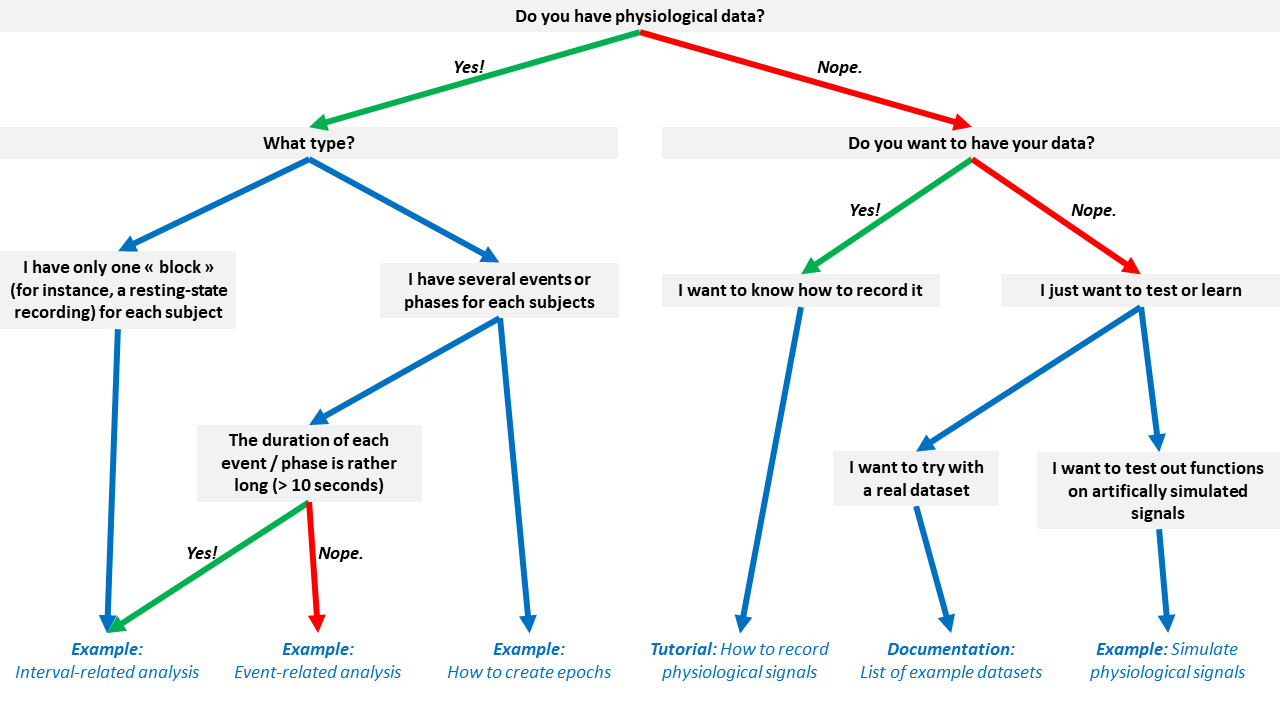## Citation¶The NeuroKit2 paper can be found here 🎉 Additionally, you can get the reference directly from Python by running:

```nk.cite()
```
```You can cite NeuroKit2 as follows:

- Makowski, D., Pham, T., Lau, Z. J., Brammer, J. C., Lespinasse, F., Pham, H.,
Schölzel, C., & Chen, S. A. (2021). NeuroKit2: A Python toolbox for neurophysiological signal processing.
Behavior Research Methods, 53(4), 1689–1696. https://doi.org/10.3758/s13428-020-01516-y

Full bibtex reference:

@article{Makowski2021neurokit,
author = {Dominique Makowski and Tam Pham and Zen J. Lau and Jan C. Brammer and Fran{\c{c}}ois Lespinasse and Hung Pham and Christopher Schölzel and S. H. Annabel Chen},
title = {{NeuroKit}2: A Python toolbox for neurophysiological signal processing},
journal = {Behavior Research Methods},
volume = {53},
number = {4},
pages = {1689--1696},
publisher = {Springer Science and Business Media {LLC}},
doi = {10.3758/s13428-020-01516-y},
url = {https://doi.org/10.3758%2Fs13428-020-01516-y},
year = 2021,
month = {feb}
}
```

Let us know if you used NeuroKit2 in a publication! Open a new discussion (select the NK in publications category) and link the paper. The community would be happy to know about how you used it and learn about your research. We could also feature it once we have a section on the website for papers that used the software.

## Physiological Data Preprocessing¶

### Simulate physiological signals¶

```import numpy as np
import pandas as pd
import neurokit2 as nk

# Generate synthetic signals
ecg = nk.ecg_simulate(duration=10, heart_rate=70)
ppg = nk.ppg_simulate(duration=10, heart_rate=70)
rsp = nk.rsp_simulate(duration=10, respiratory_rate=15)
eda = nk.eda_simulate(duration=10, scr_number=3)
emg = nk.emg_simulate(duration=10, burst_number=2)

# Visualise biosignals
data = pd.DataFrame({"ECG": ecg,
"PPG": ppg,
"RSP": rsp,
"EDA": eda,
"EMG": emg})
nk.signal_plot(data, subplots=True)
```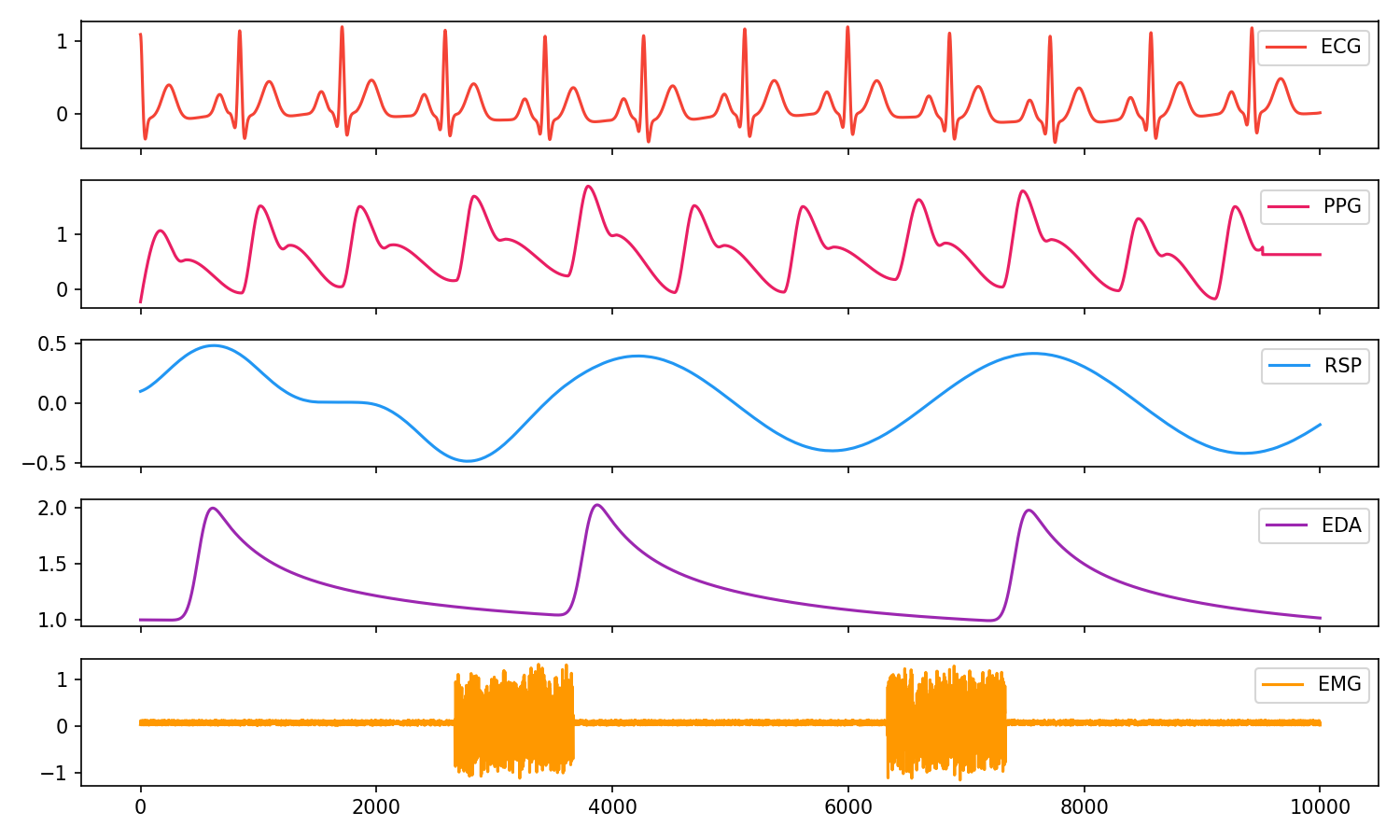### Electrodermal Activity (EDA/GSR)¶

```# Generate 10 seconds of EDA signal (recorded at 250 samples / second) with 2 SCR peaks
eda = nk.eda_simulate(duration=10, sampling_rate=250, scr_number=2, drift=0.01)

# Process it
signals, info = nk.eda_process(eda, sampling_rate=250)

# Visualise the processing
nk.eda_plot(signals, sampling_rate=250)
```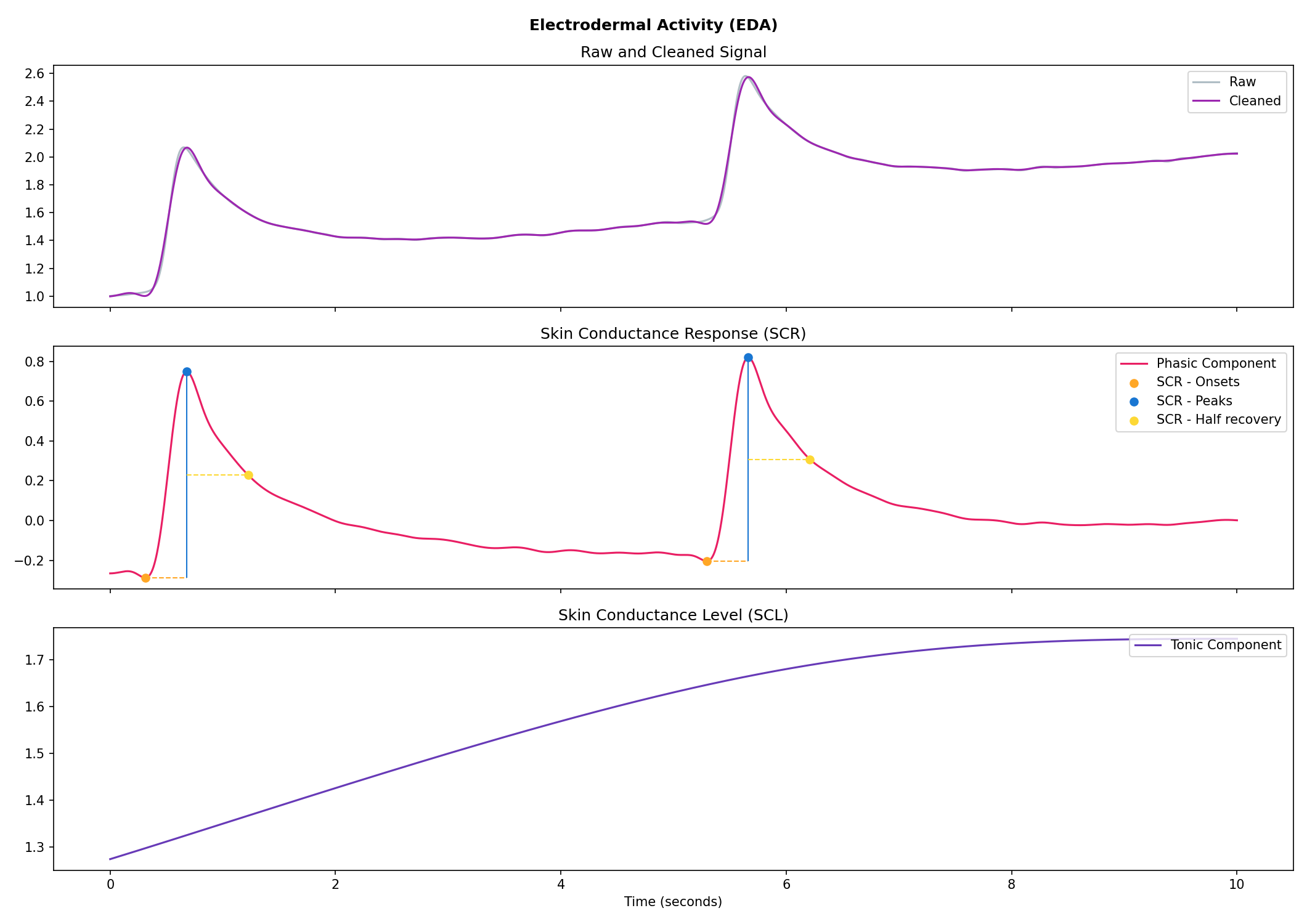### Cardiac activity (ECG)¶

```# Generate 15 seconds of ECG signal (recorded at 250 samples / second)
ecg = nk.ecg_simulate(duration=15, sampling_rate=250, heart_rate=70)

# Process it
signals, info = nk.ecg_process(ecg, sampling_rate=250)

# Visualise the processing
nk.ecg_plot(signals, sampling_rate=250)
```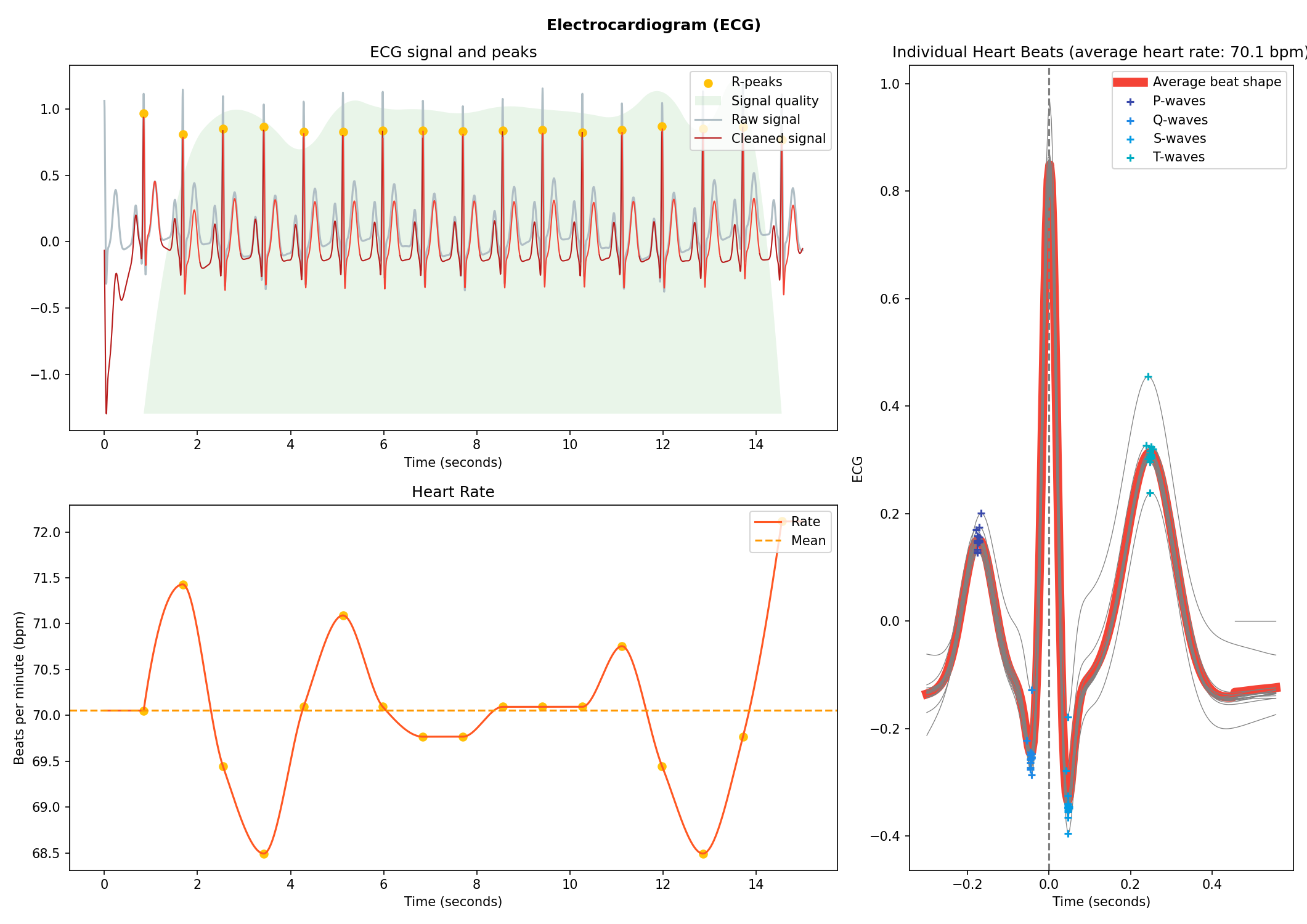### Respiration (RSP)¶

```# Generate one minute of respiratory (RSP) signal (recorded at 250 samples / second)
rsp = nk.rsp_simulate(duration=60, sampling_rate=250, respiratory_rate=15)

# Process it
signals, info = nk.rsp_process(rsp, sampling_rate=250)

# Visualise the processing
nk.rsp_plot(signals, sampling_rate=250)
```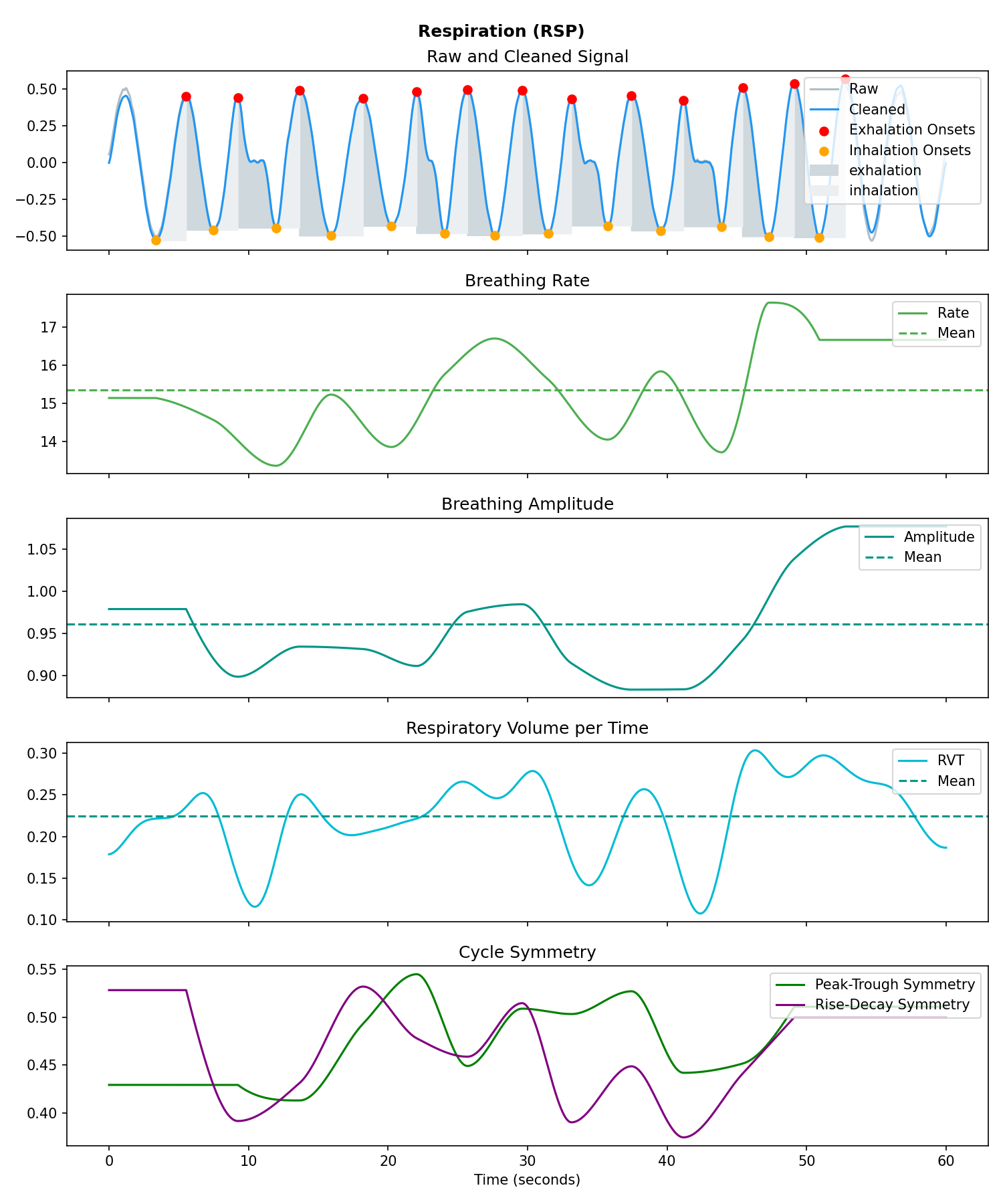### Electromyography (EMG)¶

```# Generate 10 seconds of EMG signal (recorded at 250 samples / second)
emg = nk.emg_simulate(duration=10, sampling_rate=250, burst_number=3)

# Process it
signal, info = nk.emg_process(emg, sampling_rate=250)

# Visualise the processing
nk.emg_plot(signals, sampling_rate=250)
```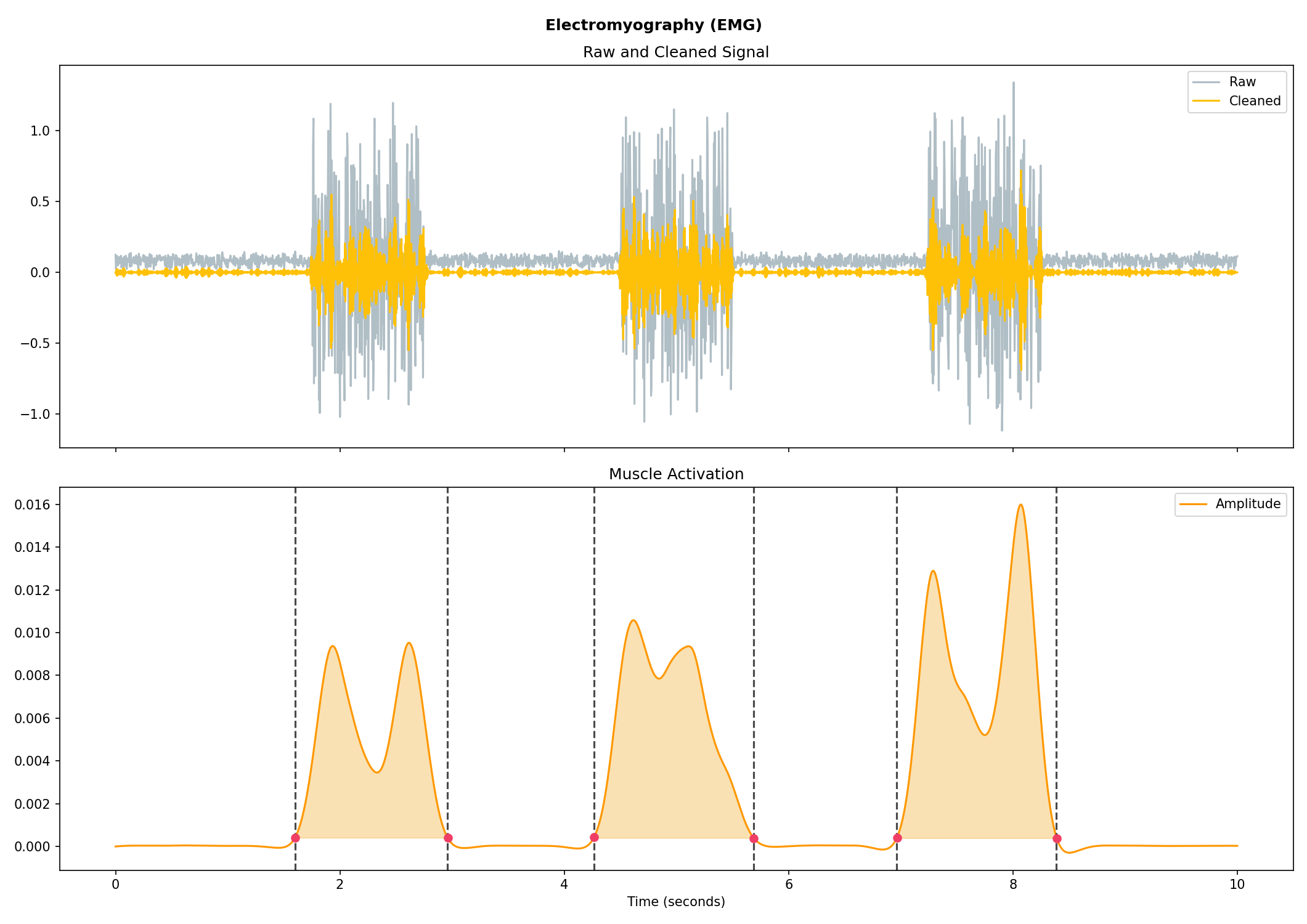### Photoplethysmography (PPG/BVP)¶

```# Generate 15 seconds of PPG signal (recorded at 250 samples / second)
ppg = nk.ppg_simulate(duration=15, sampling_rate=250, heart_rate=70)

# Process it
signals, info = nk.ppg_process(ppg, sampling_rate=250)

# Visualize the processing
nk.ppg_plot(signals, sampling_rate=250)
```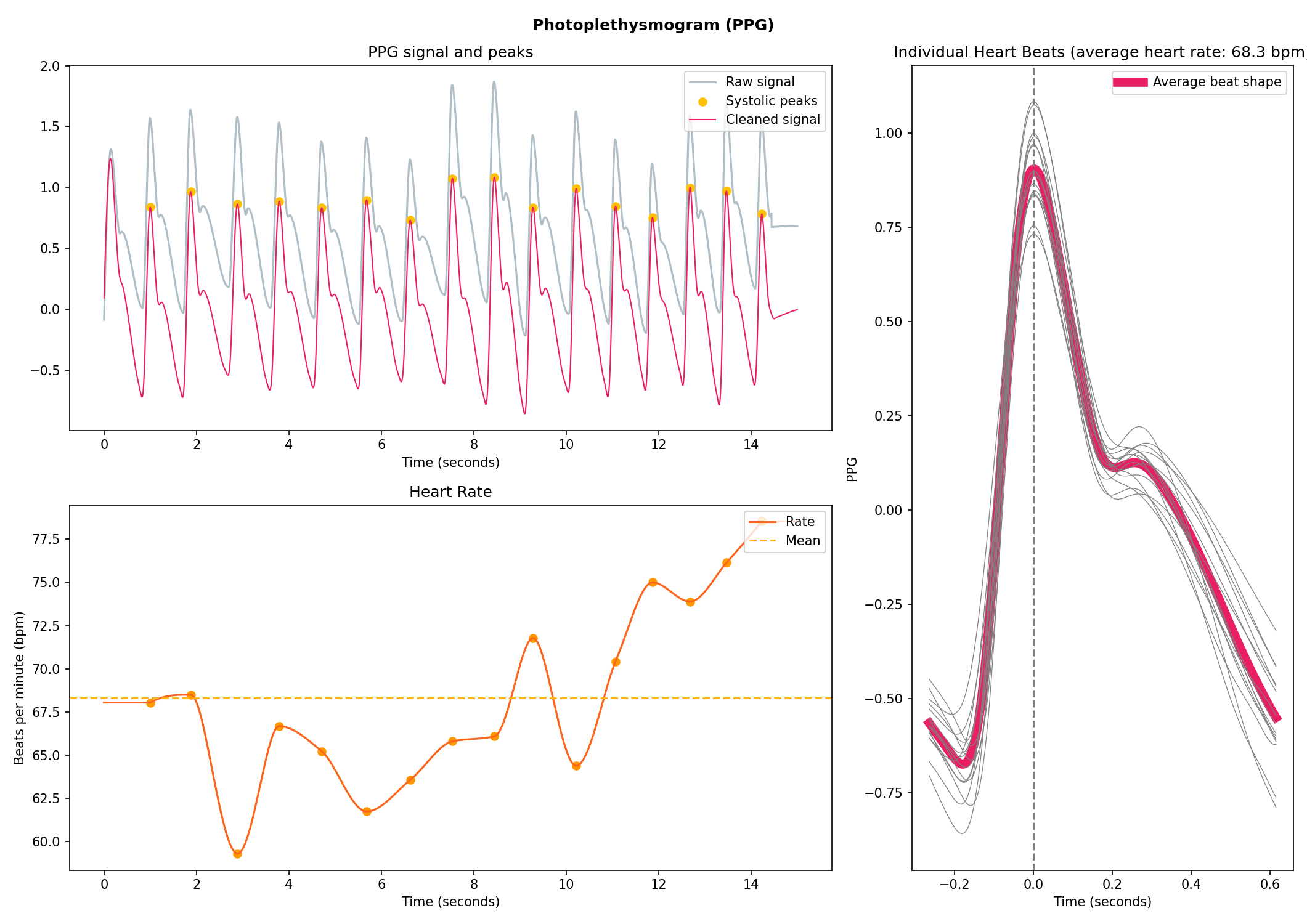### Electrooculography (EOG)¶

```# Import EOG data
eog_signal = nk.data("eog_100hz")

# Process it
signals, info = nk.eog_process(eog_signal, sampling_rate=100)

# Plot
plot = nk.eog_plot(signals, info, sampling_rate=100)
```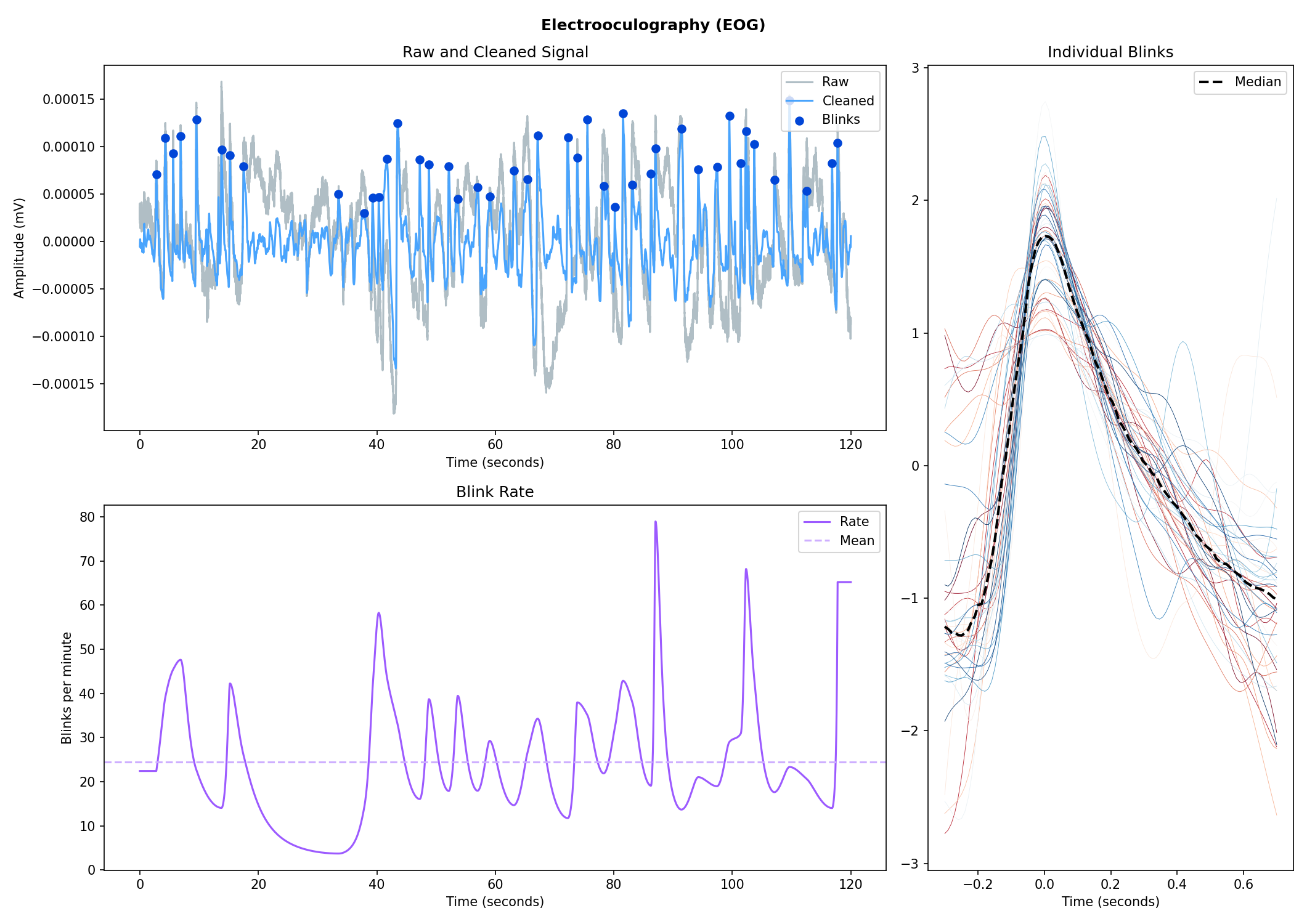### Electrogastrography (EGG)¶

Consider helping us develop it!

## Physiological Data Analysis¶

The analysis of physiological data usually comes in two types, event-related or interval-related.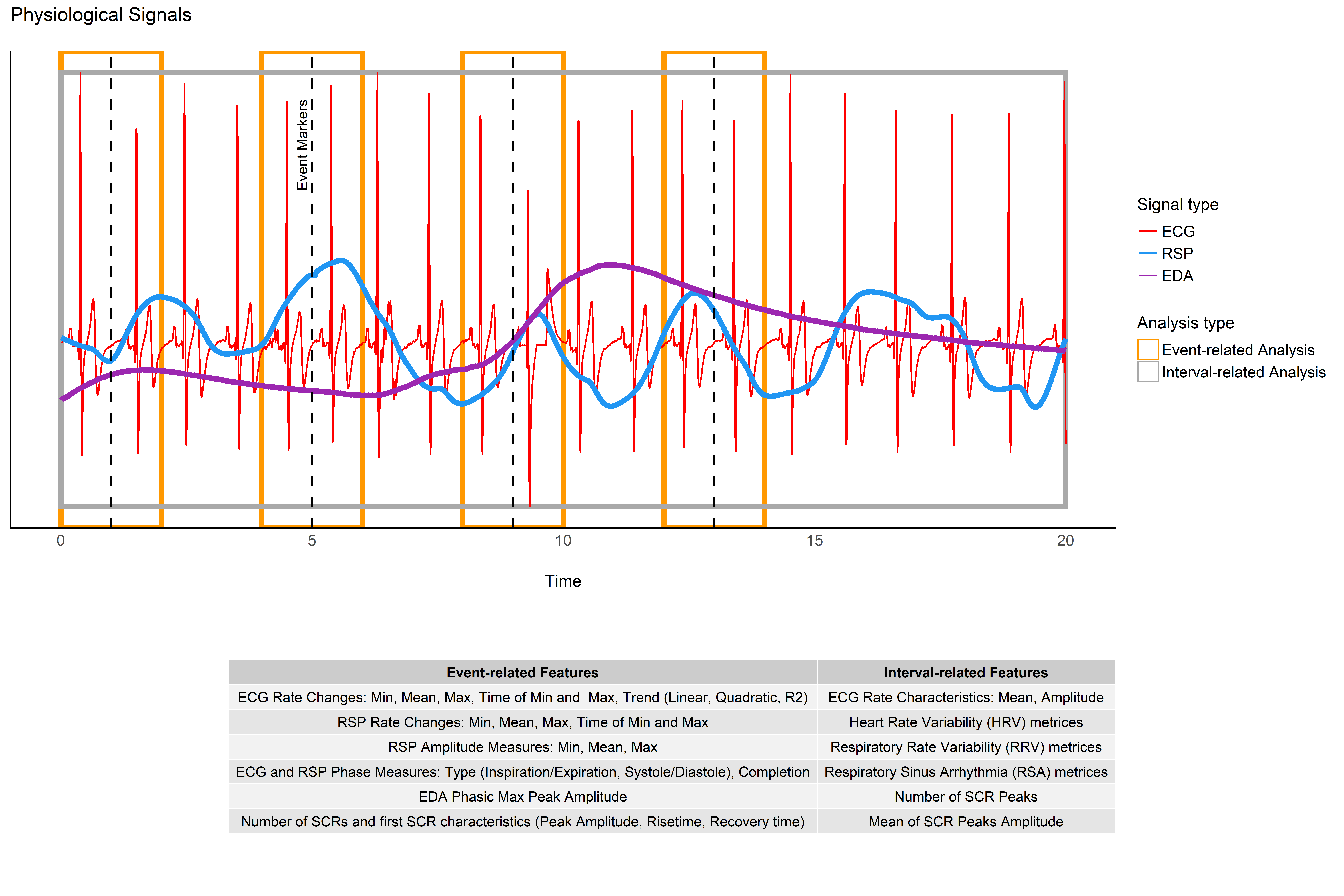## Heart Rate Variability (HRV)¶Check-out our Heart Rate Variability in Psychology: A Review of HRV Indices and an Analysis Tutorial paper for:

• a comprehensive review of the most up-to-date HRV indices

• a discussion of their significance in psychological research and practices

• a step-by-step guide for HRV analysis using NeuroKit2

```You can cite the paper as follows:

- Pham, T., Lau, Z. J., Chen, S. H. A., & Makowski, D. (2021).
Heart Rate Variability in Psychology: A Review of HRV Indices and an Analysis Tutorial.
Sensors, 21(12), 3998. https://doi:10.3390/s21123998
```
• Compute HRV indices using Python

• Time domain: RMSSD, MeanNN, SDNN, SDSD, CVNN etc.

• Frequency domain: Spectral power density in various frequency bands (Ultra low/ULF, Very low/VLF, Low/LF, High/HF, Very high/VHF), Ratio of LF to HF power, Normalized LF (LFn) and HF (HFn), Log transformed HF (LnHF).

• Nonlinear domain: Spread of RR intervals (SD1, SD2, ratio between SD2 to SD1), Cardiac Sympathetic Index (CSI), Cardial Vagal Index (CVI), Modified CSI, Sample Entropy (SampEn).

```# Download data
data = nk.data("bio_resting_8min_100hz")

# Find peaks
peaks, info = nk.ecg_peaks(data["ECG"], sampling_rate=100)

# Compute HRV indices
nk.hrv(peaks, sampling_rate=100, show=True)
>>>    HRV_RMSSD  HRV_MeanNN   HRV_SDNN  ...   HRV_CVI  HRV_CSI_Modified  HRV_SampEn
>>> 0  69.697983  696.395349  62.135891  ...  4.829101        592.095372    1.259931
```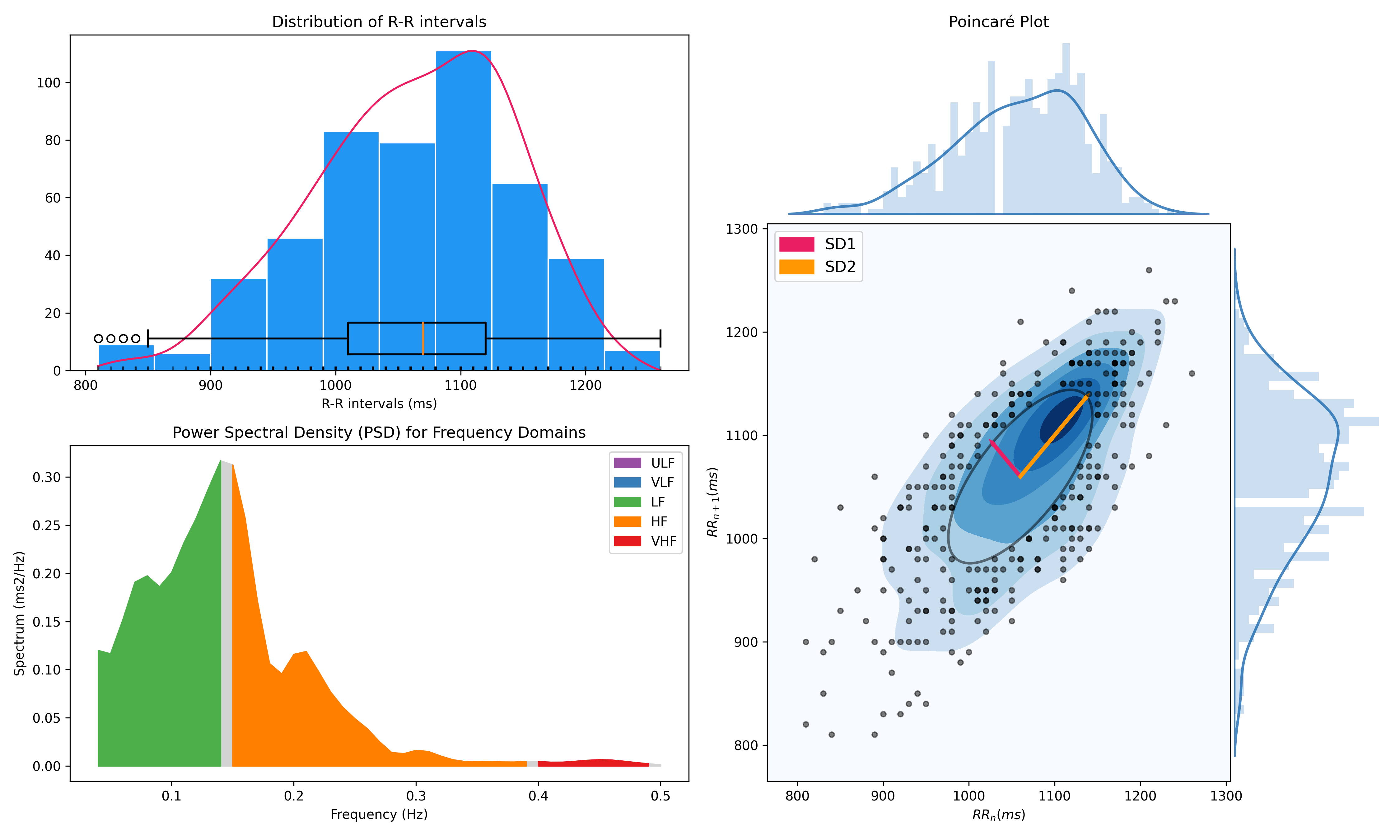## Miscellaneous¶

### ECG Delineation¶

• Delineate the QRS complex of an electrocardiac signal (ECG) including P-peaks, T-peaks, as well as their onsets and offsets.

```# Download data
ecg_signal = nk.data(dataset="ecg_3000hz")['ECG']

# Extract R-peaks locations
_, rpeaks = nk.ecg_peaks(ecg_signal, sampling_rate=3000)

# Delineate
signal, waves = nk.ecg_delineate(ecg_signal, rpeaks, sampling_rate=3000, method="dwt", show=True, show_type='all')
```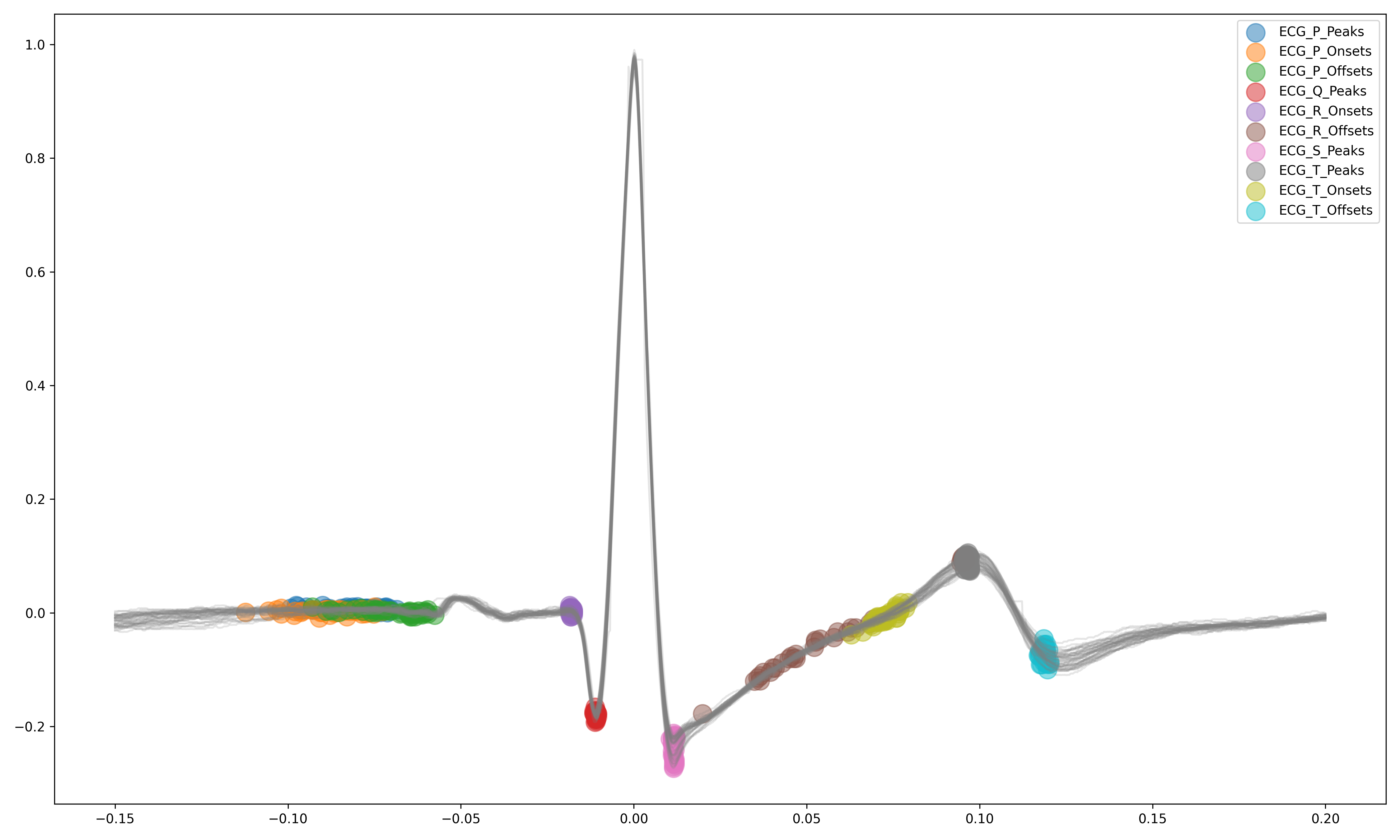### Signal Processing¶

• Signal processing functionalities

• Filtering: Using different methods.

• Detrending: Remove the baseline drift or trend.

• Distorting: Add noise and artifacts.

```# Generate original signal
original = nk.signal_simulate(duration=6, frequency=1)

# Distort the signal (add noise, linear trend, artifacts etc.)
distorted = nk.signal_distort(original,
noise_amplitude=0.1,
noise_frequency=[5, 10, 20],
powerline_amplitude=0.05,
artifacts_amplitude=0.3,
artifacts_number=3,
linear_drift=0.5)

# Clean (filter and detrend)
cleaned = nk.signal_detrend(distorted)
cleaned = nk.signal_filter(cleaned, lowcut=0.5, highcut=1.5)

# Compare the 3 signals
plot = nk.signal_plot([original, distorted, cleaned])
```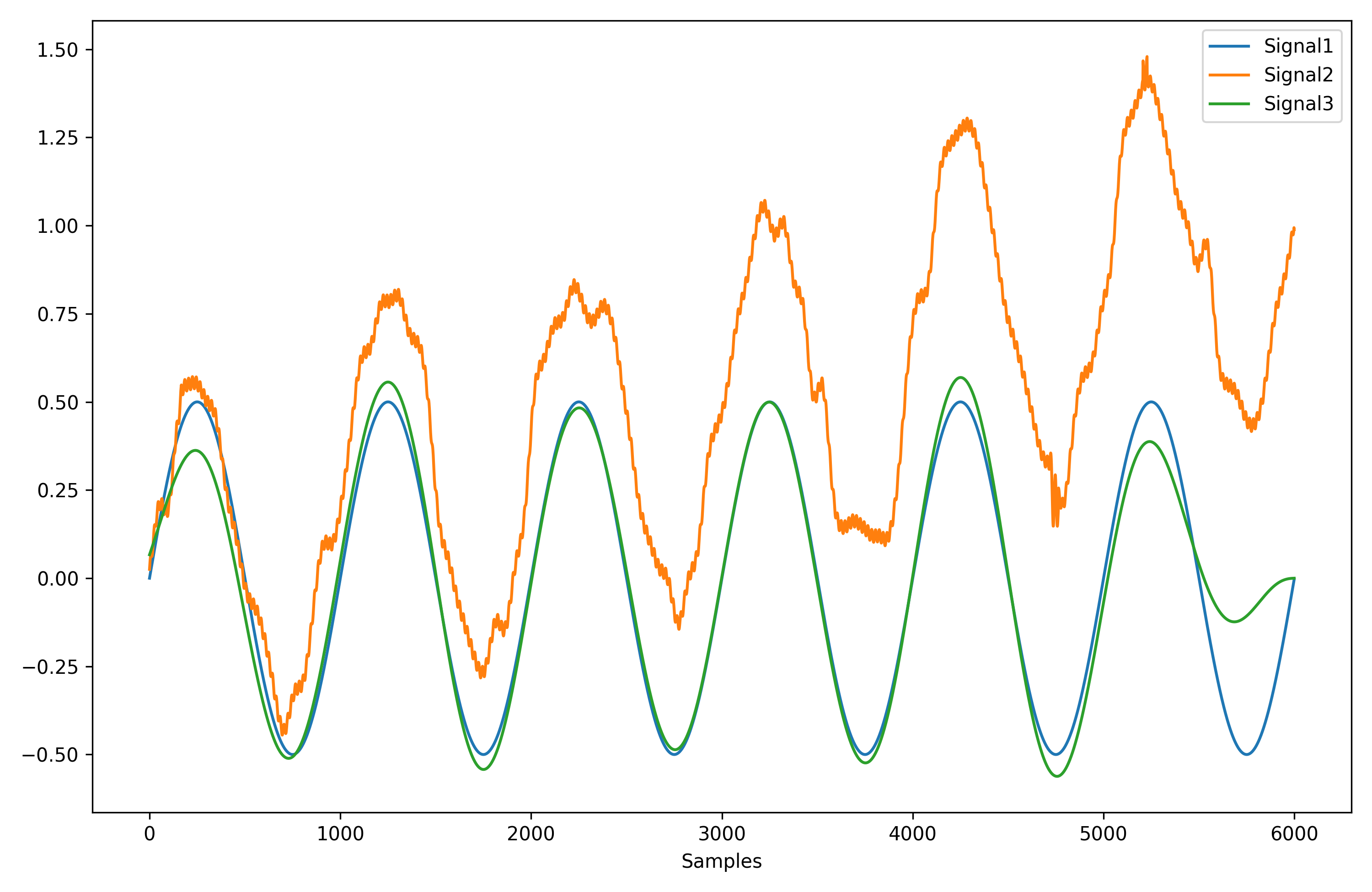### Complexity (Entropy, Fractal Dimensions, …)¶

• Optimize complexity parameters (delay tau, dimension m, tolerance r)

```# Generate signal
signal = nk.signal_simulate(frequency=[1, 3], noise=0.01, sampling_rate=100)

# Find optimal time delay, embedding dimension and r
parameters = nk.complexity_optimize(signal, show=True)
```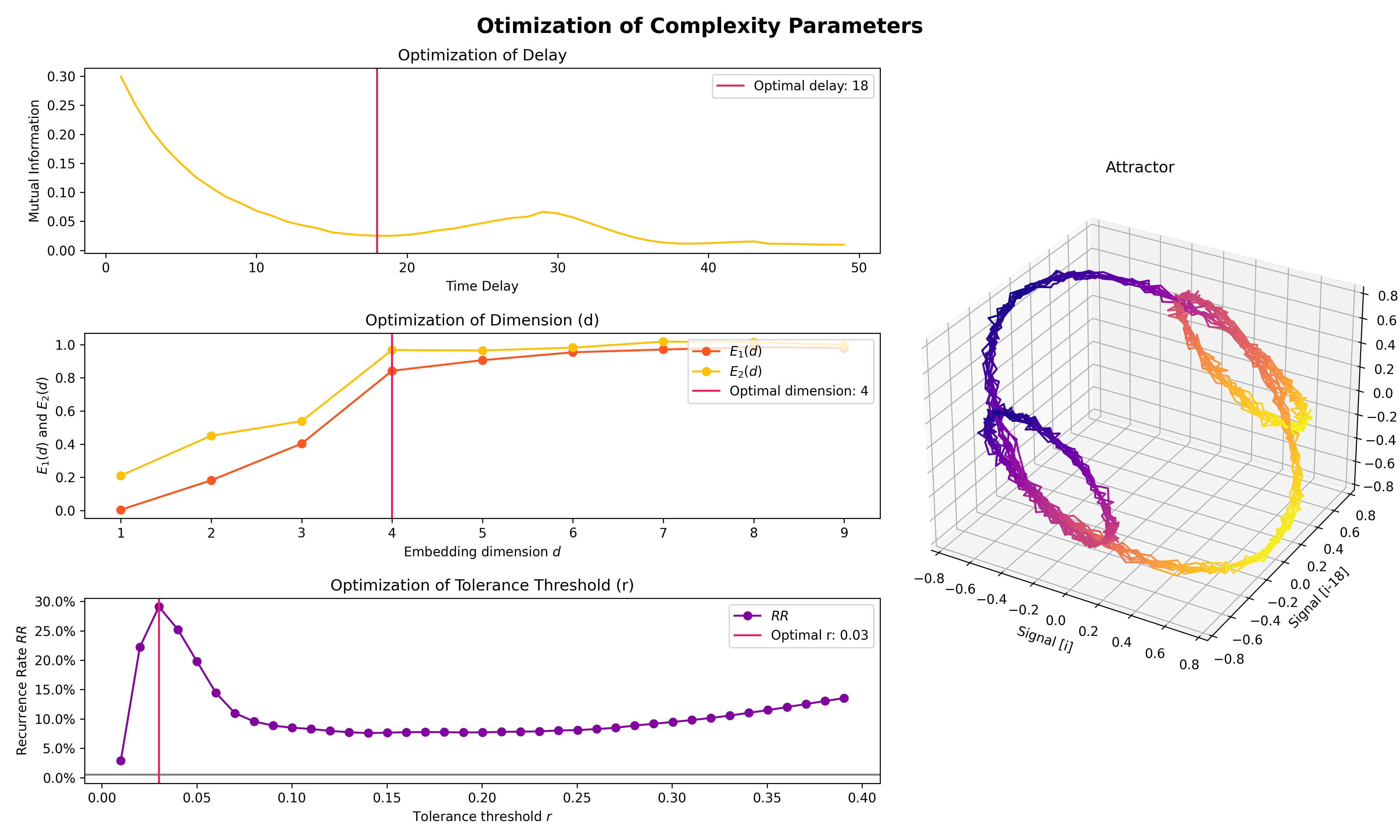• Compute complexity features

• Entropy: Sample Entropy (SampEn), Approximate Entropy (ApEn), Fuzzy Entropy (FuzzEn), Multiscale Entropy (MSE), Shannon Entropy (ShEn)

• Fractal dimensions: Correlation Dimension D2, …

• Detrended Fluctuation Analysis

```nk.entropy_sample(signal)
nk.entropy_approximate(signal)
```

### Signal Decomposition¶

```# Create complex signal
signal = nk.signal_simulate(duration=10, frequency=1)  # High freq
signal += 3 * nk.signal_simulate(duration=10, frequency=3)  # Higher freq
signal += 3 * np.linspace(0, 2, len(signal))  # Add baseline and linear trend
signal += 2 * nk.signal_simulate(duration=10, frequency=0.1, noise=0)  # Non-linear trend
signal += np.random.normal(0, 0.02, len(signal))  # Add noise

# Decompose signal using Empirical Mode Decomposition (EMD)
components = nk.signal_decompose(signal, method='emd')
nk.signal_plot(components)  # Visualize components

# Recompose merging correlated components
recomposed = nk.signal_recompose(components, threshold=0.99)
nk.signal_plot(recomposed)  # Visualize components
```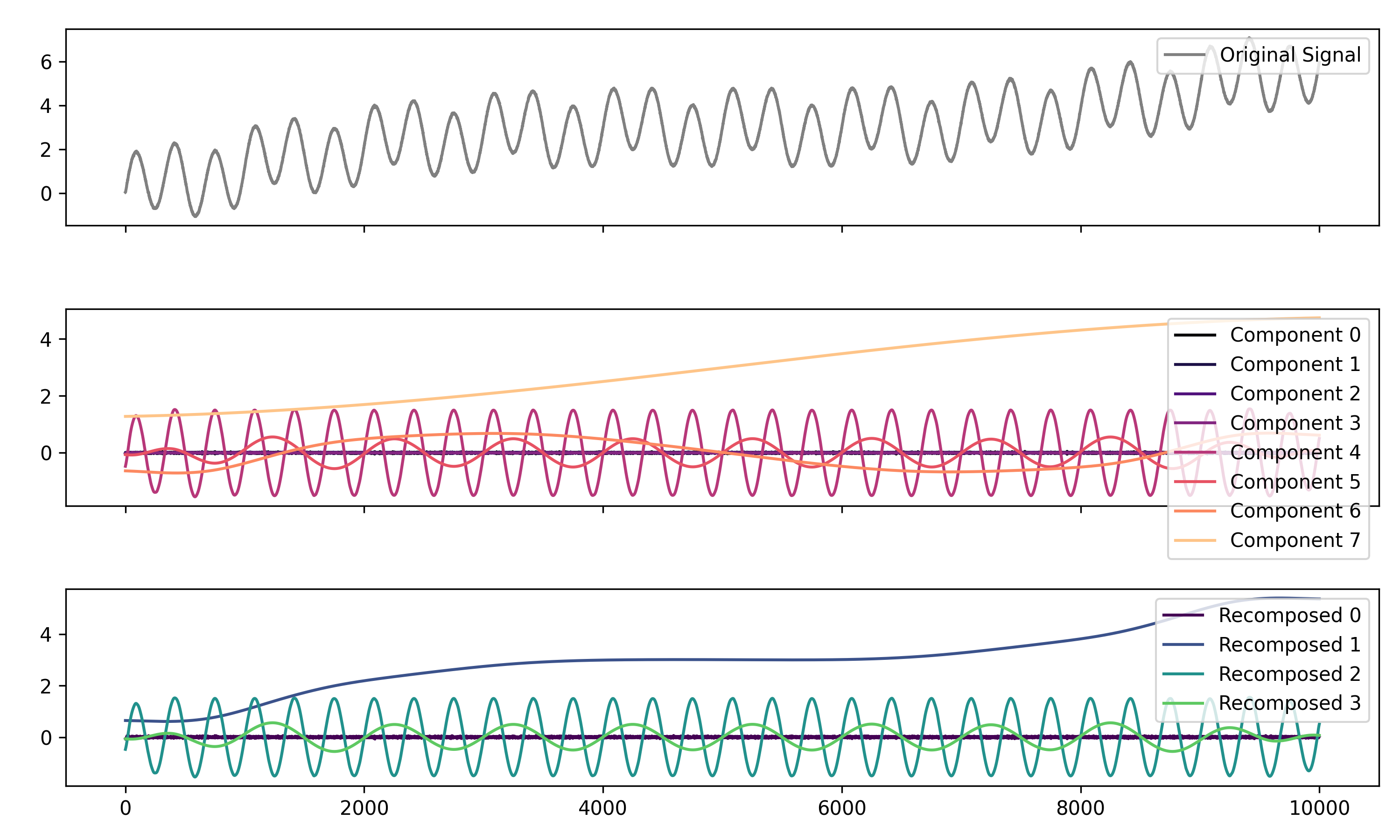### Signal Power Spectrum Density (PSD)¶

```# Generate complex signal
signal = nk.signal_simulate(duration=20, frequency=[0.5, 5, 10, 15], amplitude=[2, 1.5, 0.5, 0.3], noise=0.025)

# Get the PSD using different methods
welch = nk.signal_psd(signal, method="welch", min_frequency=1, max_frequency=20, show=True)
multitaper = nk.signal_psd(signal, method="multitapers", max_frequency=20, show=True)
lomb = nk.signal_psd(signal, method="lomb", min_frequency=1, max_frequency=20, show=True)
burg = nk.signal_psd(signal, method="burg", min_frequency=1, max_frequency=20, order=10, show=True)
```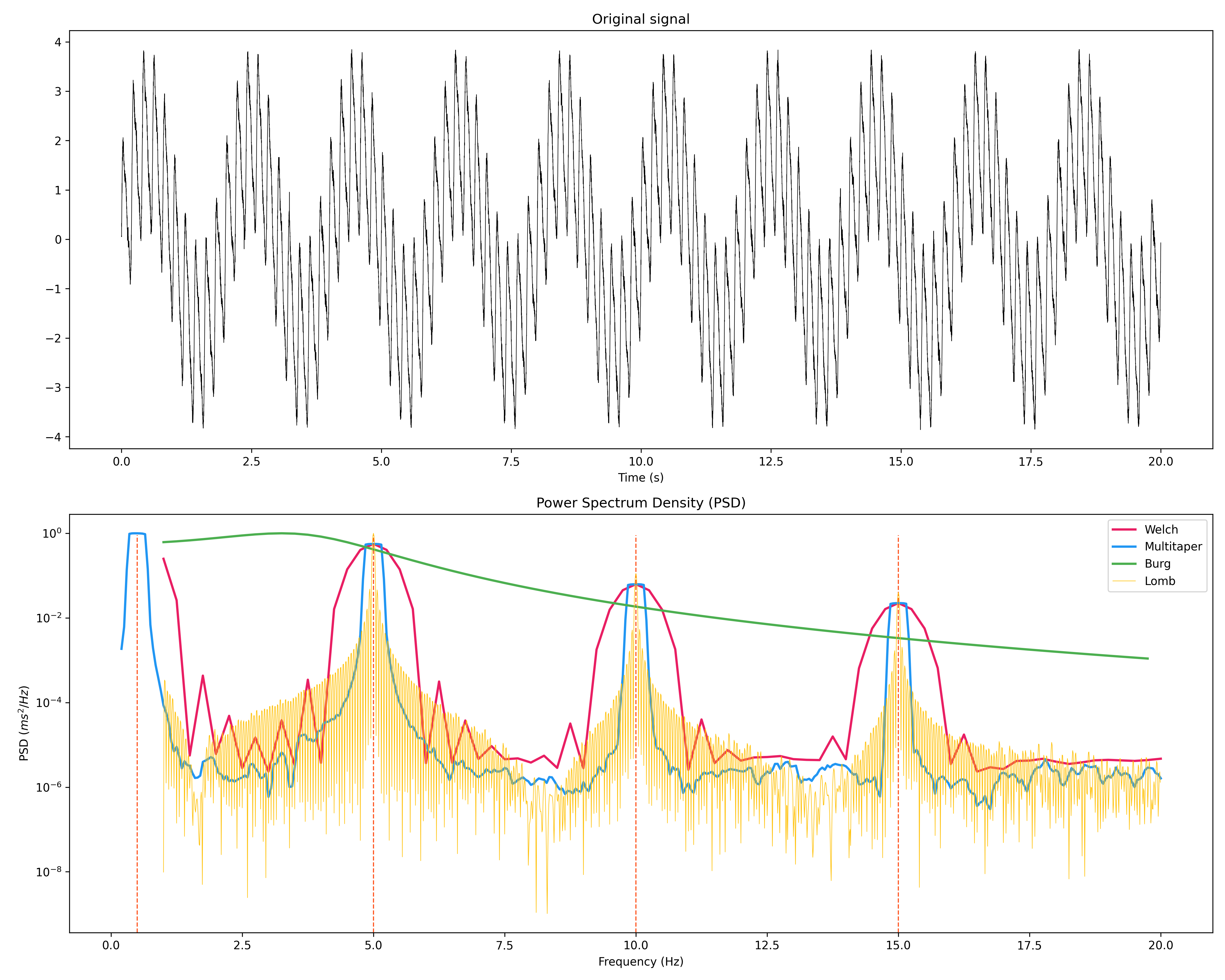### Statistics¶

• Highest Density Interval (HDI)

```x = np.random.normal(loc=0, scale=1, size=100000)

ci_min, ci_max = nk.hdi(x, ci=0.95, show=True)
```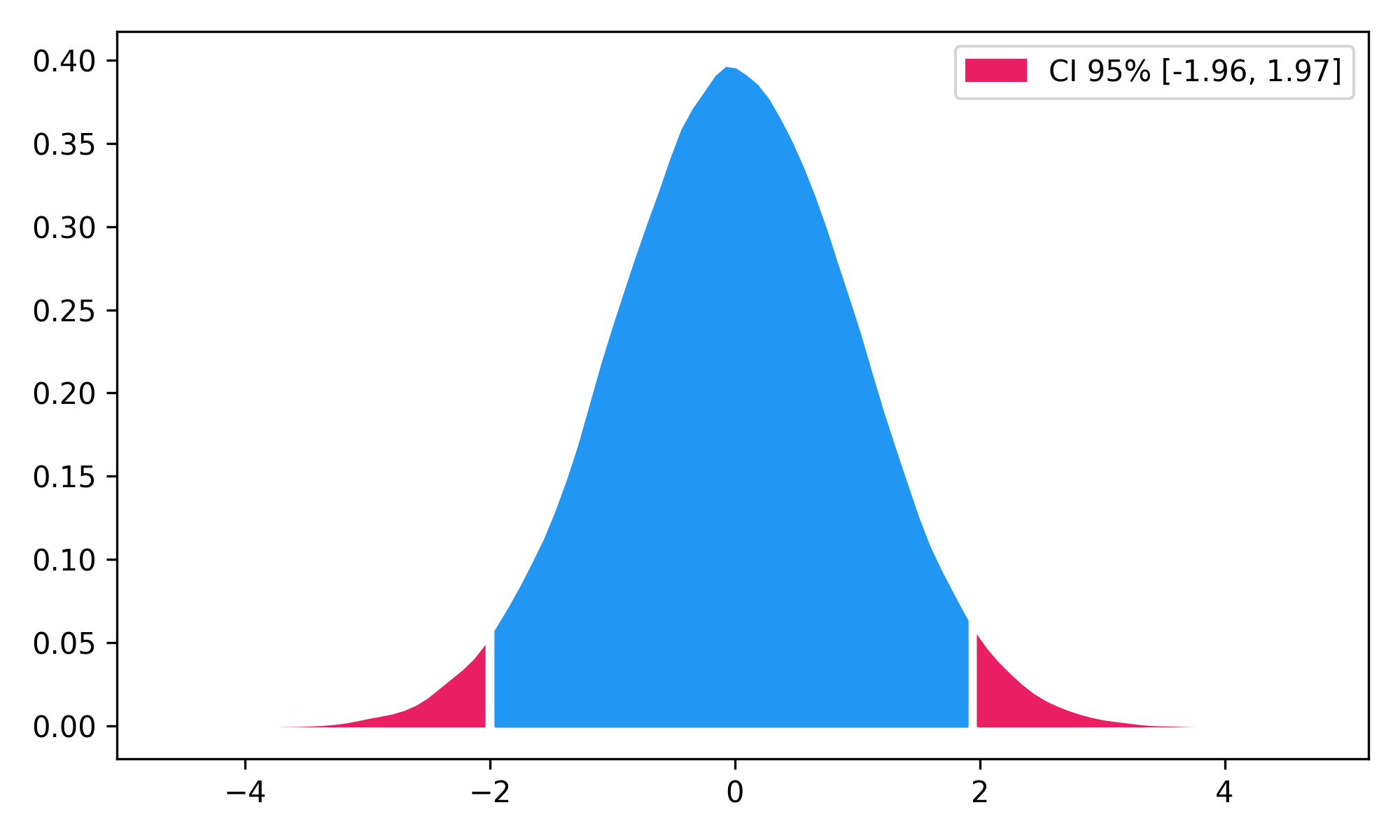## Popularity¶NeuroKit2 is one of the most welcoming package for new contributors and users, as well as the fastest growing package. So stop hesitating and hop onboard 🤗